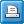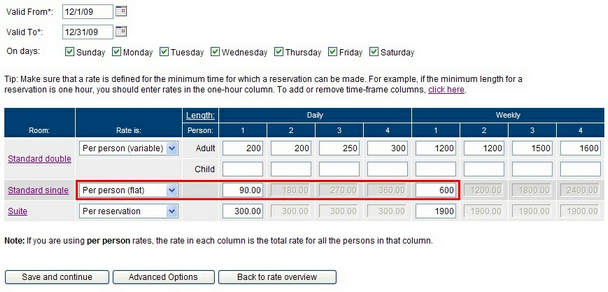﻿ Rates: Per person (flat)
WebReserv User Guide

Rates: Per person (flat)

 Rates: Per person (flat)Rates: Per person (flat)If the rate is per person (flat), the booking system will use the same rate per person. To calculate the total rate, the system will multiply the rate with the number of persons on the reservation and the duration of the reservation.

Number of persons x number of days x flat rate = total rate

Example: If the rate is set to per person (flat) with a daily of 90.00 and a reservation is made for 2 persons for 3 days, the total rate will be

2 (persons) x 3 (days) x 90 = 540Back to edit rate list